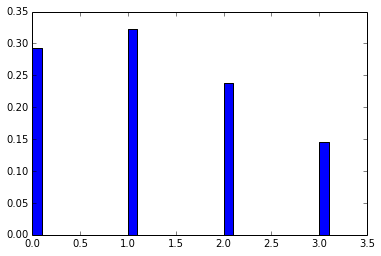Chapter 5 : principles of statistical thermodynamics¶

Example 5.1 pg : 104¶

In :
from  math import factorial

# Variables
N1 = 1.
N2 = 1.
N3 = 3.
N4 = 1.

# Calculations
N = N1+N2+N3+N4
sig = factorial(N) /(factorial(N1) *factorial(N2)*factorial(N3)*factorial(N4))

# Results
print "No. of ways of arranging  =  %d "%(sig)
No. of ways of arranging  =  120

Example 5.2 pg : 104¶

In :

# Variables
N = 6.
g = 4.

# Calculations
sig = factorial(g+N-1) /(factorial(g-1) *factorial(N))

# Results
print "No. of ways of arranging  =  %d "%(sig)
No. of ways of arranging  =  84

Example 5.3 pg : 104¶

In :
from math import factorial
# Variables
N = 6.
g = 8.

# Calculations
sig = factorial(g) /(factorial(N) *factorial(g-N))

# Results
print "No. of ways   =  %d "%(sig)
No. of ways   =  28

Example 5.4 pg : 121¶

In :
%matplotlib inline

import math
from matplotlib.pyplot import bar

# Variables
N0 = 1.

# Calculations
N1 = 3/math.e
N2 = 6/math.e**2
N3 = 10/math.e**3
N = N0+N1+N2+N3
ei = [0, 1, 2, 3]

f0 = N0/N
f1 = N1/N
f2 = N2/N
f3 = N3/N
fi = [f0, f1, f2, f3]

# Results
print "fractional population of level 0  =  %.3f"%(f0)
print "  fractional population of level 1  =  %.3f"%(f1)
print "  fractional population of level 2  =  %.3f"%(f2)
print "  fractional population of level 3  =  %.3f"%(f3)
bar(ei,fi,0.1)
fractional population of level 0  =  0.293
fractional population of level 1  =  0.323
fractional population of level 2  =  0.238
fractional population of level 3  =  0.146
Out:
<Container object of 4 artists>